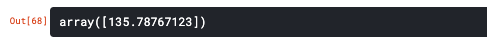top of pageSearch

# Linear Regression - Quick Look

Linear regression is a statistical model to understand the relationship between the dependent and the independent variable.Linear Regression helps to predict the relationship between the two variables. For eg say, the relation between the sales and the promotion spending can be predicted .Thus , it helps to understand how much the dependent variable changes when one or more independent variable change.Let’s dig real quick to understand how Linear Regression is implemented with an example.

In this example ,we are going to predict the sale of the house in an area based on its square feet value.Take a closure look on the below scatter plotHere, X-axis represents the Square feet and the Y axis represents the Price .Once we derive the best fit line (that connects most of the data points)based on the Linear Regression equation , we can easily predict the price of an house with 3300 sqft as roughly around 628700

Simple Linear Equation is derived from the below formulaHere, y is the dependent variable ,m is the gradient or slope , x is the independent variable and b is the y-intercept.

In our case, it will be Price = m * Square feet + b

Step 1 : Import all the necessary libraries.

```import numpy as np
import pandas as pd
import matplotlib.pyplot as plt
import seaborn as sns
from sklearn.model_selection import train_test_split
from sklearn.linear_model import LinearRegression```

`data=pd.read_csv('/kaggle/input/house-price/House Price Prediction.csv')`
`data`

OutputStep 3: We are plotting a scatter plot , just to get an idea about data points.

```plt.figure(figsize=(12,6))
sns.pairplot(data,x_vars=[‘Square feet’],y_vars=[‘Price’],size=7,kind=’scatter’)
plt.xlabel(‘Square Feet’)
plt.ylabel(‘Price’)
plt.title(‘House Price Prediction’)
plt.show()```

OutputStep 4:Linear Regression object is created and we are parsing our available data (the square feet(X axis) and the price(Y axis)

```lr = LinearRegression()
lr.fit(data[[‘Square feet’]],data.Price)```

OutputStep 5: If suppose, you want to predict the price of a house with squarefeet 3300 , it calculates as =>628715.75

`lr.predict([])`

OutputAhh !! Wait how does it calculated !!! Hmm..Let’s travel back to the equation . y=mx+b, here the slope m is calculated with the help of coef_ and b, the intercept is calculated using intercept_

`lr.coef_`

Output`lr.intercept_`

OutputSo now ,in our case it will be like

price = m * squarefeet + b

`135.78767123 * 3300 + 180616.43835`

OutputNow it is very clear how the square feet 3300 was predicted in Step 5 .

Conclusion -

This context vividly explains how the values are calculated using the linear regression model .Large chuck's of data can be parsed in as an array and the values can be predicted instead of parsing as single value as shown in the above example